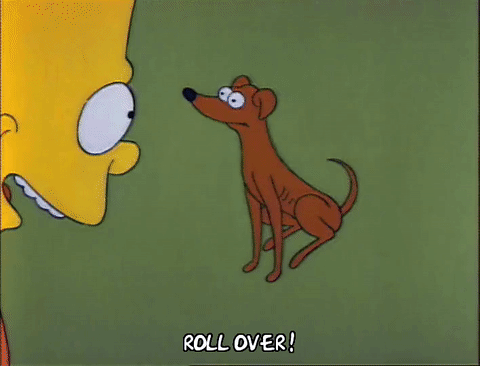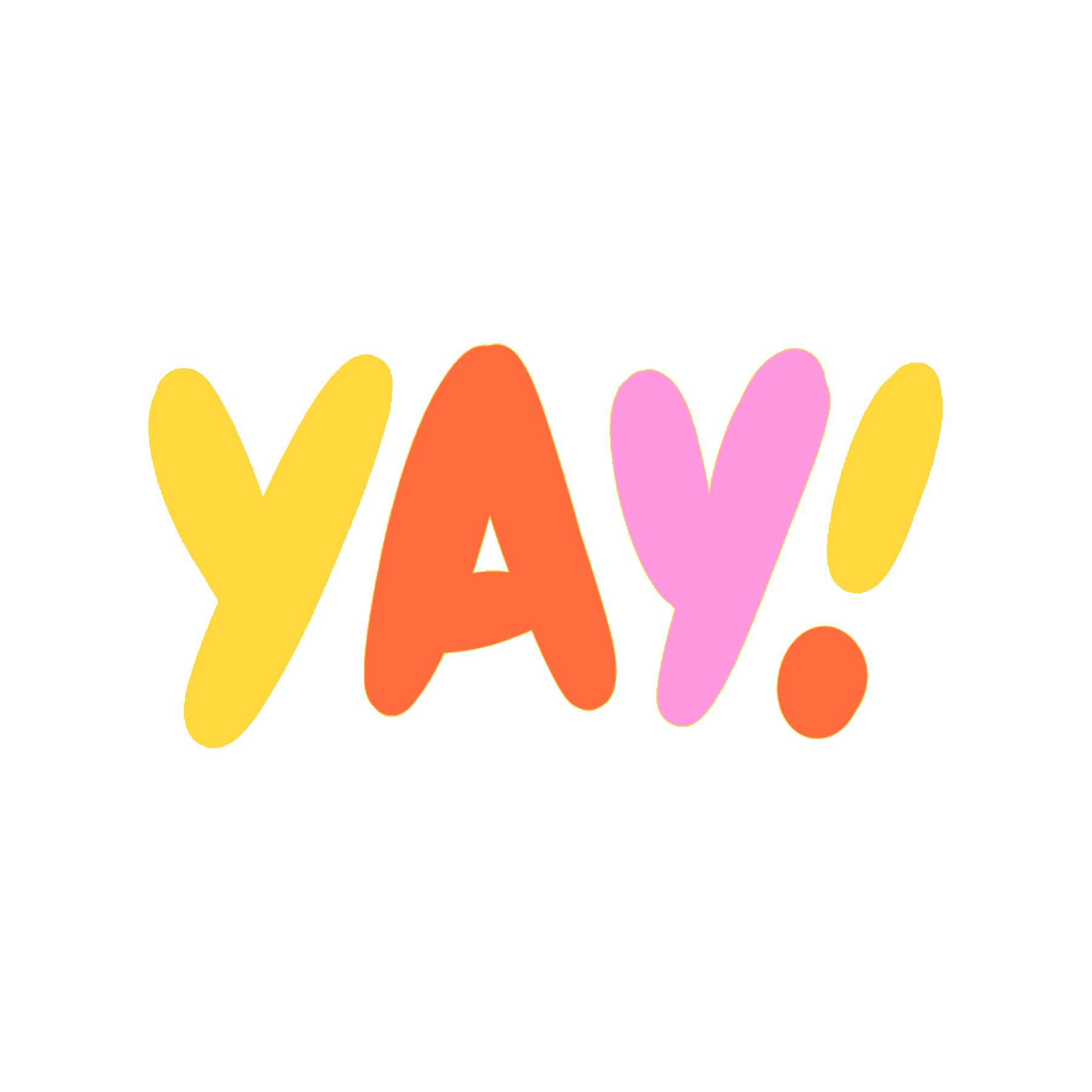# Dice Rolling

## Create a dice rolling simulator with Python and turtle!@JakeGerber

Create the dice rolling simulation of your dreams with this workshop by using Python and the turtle library. It's cool even if you don't play DND! Maybe you just like rolling dice?Final demo and code

## Getting started

We're going to use Repl.it, a free, online coding environment, to create the project.

Create a new Python repl by going to repl.it/languages/python3## Initial StatementsTime to start coding! Let's begin by importing the two libaries we'll need. Add this code to the `main.py` file:

``````import dice
import turtle
``````
• `dice` in this case does not refer to the Python library; it's importing the functions from a file called `dice.py`, which we'll write in a minute
• `turtle` is a Python library that makes it super easy to create visuals

``````import dice
import turtle

reroll()

turtle.onkey(reroll, "space")

turtle.listen()
turtle.mainloop()
``````

We are calling a function called `reroll()`, which we will create in a moment. Then, if the space key is pressed, the reroll function is called, and the turtle is always listening for user input.

## RerollLet's write the `reroll()` function.

``````import dice
import turtle

def reroll():

# Code we just wrote
``````

Add this just after the `import` statements, but before the rest of the code that we just wrote.

``````def reroll():
turtle.clear()
turtle.tracer(0, 0)
turtle.update()
``````
• First, we clear the screen so that we can draw new dice.
• Then, we add turtle's `tracer()` and `update()` functions. These make it so that the dice we draw are fully drawn at the time time, instead of the screen refreshing after every draw call. Basically, without these statements, the dice would draw VERY slowly.
``````def reroll():
turtle.clear()
turtle.tracer(0, 0)
dice.Die(0, 0, 100, 10, "black", "red")

dice.Die(0, 150, 100, 10, "black", "red")

dice.Die(0, -150, 100, 10, "black", "red")
turtle.update()
``````

The `dice.py` file that we imported earlier and will create in a moment is used here to draw the Die function. This will make sense in a moment!

## Creating the `dice.py` file

Create the `dice.py` file as shown below. We're gonna put all of our fancy dice functions in this file.``````import turtle
import random
``````

For the rest of the workshop, all code will be written in `dice.py` unless specified otherwise.

Add these statements to the top so we can access the `turtle` and `random` libraries. The `random` library allows us to generate random numbers.

## Drawing the BoxEvery dice has a box. If not then it's just circles. Only circles. Pretty ominous. Let's add a function that draws a box.

``````import turtle
import random

def drawBox(x, y, size, color):
``````

This function will draw the box of the dice. It takes in x and y coordinates, a size, and a color.

``````def drawBox(x, y, size, color):
turtle.color(color)
turtle.up()
turtle.goto(x, y)
``````

Inside the `drawBox()` function, we are setting the turtle's color to the parameter color, setting the direction, making the turtle go up, and going to the coordinates.

``````def drawBox(x, y, size, color):
turtle.color(color)
turtle.up()
turtle.goto(x, y)
turtle.begin_fill()
turtle.end_fill()
``````

Add the `begin_fill()` and `end_fill()` statements so the box drawn will be filled in.

``````def drawBox(x, y, size, color):
turtle.color(color)
turtle.up()
turtle.goto(x, y)
turtle.begin_fill()
for x in range(4):
turtle.forward(size)
turtle.right(90)
turtle.end_fill()
``````

Then, add a for loop and draw the box. We're using a for loop here so that we can draw the box on each side of the die!

## Rolling the Die``````def rolls(x, y, size, radius, color, roll):
turtle.color(color)
turtle.up()
``````

Now, under all of the code you just wrote, create a new function called `rolls()`. This function will take in x and y coordinates, the size of the box we just created, the radius of the circles, and the random number that will be passed in.

Inside the newly-created `rolls()` function, we set the color to the passed-in color, and go up.

### Row 1

Every row has three dots and there are three rows. Let's start with row 1.

``````def rolls(x, y, size, radius, color, roll):
turtle.color(color)
turtle.up()

#row 1
``````

Go to the location of the first circle in the first row of the die. The die scales depending on the box size.

``````def rolls(x, y, size, radius, color, roll):
#Previous code would be here.

#row 1

#circle 1 (row 1)
if (roll == 2 or roll == 3 or roll == 4 or roll == 5 or roll == 6):
turtle.begin_fill()
turtle.end_fill()

turtle.forward(size/3)
``````

If the roll is a 2, 3, 4, 5, or 6, then draw the first circle. We always need `begin_fill()` and `end_fill()` statements for these circles so they are filled in. Draw the circle with the radius that was passed in as the parameter, and afterwards, go forward 1/3 the size of the box.

``````def rolls(x, y, size, radius, color, roll):
#Previous code would be here.

#circle 2 (row 1)
"""
if ():
turtle.begin_fill()
turtle.end_fill()
"""

turtle.forward(size/3)
``````

There is never a case where the second circle on the first row is used, which is why it is commented out. Once again, move forward 1/3 the size of the box.

``````def rolls(x, y, size, radius, color, roll):
#Previous code would be here.

#circle 3 (row 1)
if (roll == 4 or roll == 5 or roll == 6):
turtle.begin_fill()
turtle.end_fill()
``````

If the roll is 4, 5, or 6, draw the filled in circle.

## Row 2

Now onto row 2. Keep it up!

``````def rolls(x, y, size, radius, color, roll):
#Previous code would be here.

#row 2
``````

Let's go to the location of the first circle on the second row.

``````def rolls(x, y, size, radius, color, roll):
#Previous code would be here.

#circle 1 (row 2)
if (roll == 6):
turtle.begin_fill()
turtle.end_fill()

turtle.forward(size/3)
``````

If the roll is a 6, draw the circle. Then, move forward to the second circle.

``````def rolls(x, y, size, radius, color, roll):
#Previous code would be here.

#circle 2 (row 2)
if (roll == 1 or roll == 3 or roll == 5):
turtle.begin_fill()
turtle.end_fill()

turtle.forward(size/3)
``````

If the roll is a 1, 3, or 5, draw the circle. Then move forward to the third circle.

``````def rolls(x, y, size, radius, color, roll):
#Previous code would be here.

#circle 3 (row 2)
if (roll == 6):
turtle.begin_fill()
turtle.end_fill()
``````

If the roll is a 6, draw the third circle.

## Row 3

Final row to draw. Hang in there!

``````def rolls(x, y, size, radius, color, roll):
#Previous code would be here.

#row 3
``````

Go to the location of the first circle on the third row.

``````def rolls(x, y, size, radius, color, roll):
#Previous code would be here.

#circle 1
if (roll == 4 or roll == 5 or roll == 6):
turtle.begin_fill()
turtle.end_fill()

turtle.forward(size/3)
``````

If the roll is a 4, 5, or 6, then draw the circle. Then go forward to the location of the second circle.

``````def rolls(x, y, size, radius, color, roll):
#Previous code would be here.

#circle 2

"""
if ():
turtle.begin_fill()
turtle.end_fill()
"""

turtle.forward(size/3)
``````

There is no roll where the second circle is drawn so it is commented out. Move forward to the third circle.

``````def rolls(x, y, size, radius, color, roll):
#Previous code would be here.

#circle 3
if (roll == 2 or roll == 3 or roll == 4 or roll == 5 or roll == 6):
turtle.begin_fill()
turtle.end_fill()
``````

If the roll is a 2, 3, 4, 5, or 6, then draw the circle.

## Putting It All Together

We are creating a function that will call the functions to draw the box and the circles. You're so close to the end that I cannot even comprehend how close you are because you are just so close!!!

``````def Die(x, y, size, radius, color1, color2):
``````

Add this function right below the other ones we just wrote. It takes in x and y coordinates, the size of the box, the radius of the circles, and two colors (one for the box and one for the circles).

``````def Die(x, y, size, radius, color1, color2):
turtle.speed(0)
turtle.hideturtle()
x -= (size/2)
y += (size/2)
``````

The turtle's speed is set to 0 so it draws instantly, and the cursor is hidden. The x and y coordinates are repositioned because when the user inputs coordinates, we want the coordinates to be for the center of the box. Changing the x and y accounts for this.

``````def Die(x, y, size, radius, color1, color2):
turtle.speed(0)
turtle.hideturtle()
x -= (size/2)
y += (size/2)
drawBox(x, y, size, color1)
rolls(x, y, size, radius, color2, random.randint(1, 6))
``````

The `drawBox()` and `rolls()` functions are called with their respective parameters.

## You're done!

Yay! You're done! Now, if you click the green "Run" button at the top, you can see your dice in action.Here's the final code:

## main.py

``````import dice
import turtle

def reroll():
turtle.clear()
turtle.tracer(0, 0)
dice.Die(0, 0, 100, 10, "black", "red")

dice.Die(0, 150, 100, 10, "black", "red")

dice.Die(0, -150, 100, 10, "black", "red")
turtle.update()

reroll()

turtle.onkey(reroll, "space")

turtle.listen()
turtle.mainloop()
``````

## dice.py

``````import turtle
import random

def drawBox(x, y, size, color):
turtle.color(color)
turtle.up()
turtle.goto(x, y)
turtle.begin_fill()
for x in range(4):
turtle.forward(size)
turtle.right(90)
turtle.end_fill()

def rolls(x, y, size, radius, color, roll):
turtle.color(color)
turtle.up()

#row 1

#circle 1
if (roll == 2 or roll == 3 or roll == 4 or roll == 5 or roll == 6):
turtle.begin_fill()
turtle.end_fill()

turtle.forward(size/3)

#circle 2
'''
if ():
turtle.begin_fill()
turtle.end_fill()
'''

turtle.forward(size/3)

#circle 3
if (roll == 4 or roll == 5 or roll == 6):
turtle.begin_fill()
turtle.end_fill()

#row 2

#circle 1
if (roll == 6):
turtle.begin_fill()
turtle.end_fill()

turtle.forward(size/3)

#circle 2
if (roll == 1 or roll == 3 or roll == 5):
turtle.begin_fill()
turtle.end_fill()

turtle.forward(size/3)

#circle 3
if (roll == 6):
turtle.begin_fill()
turtle.end_fill()

#row 3

#circle 1
if (roll == 4 or roll == 5 or roll == 6):
turtle.begin_fill()
turtle.end_fill()

turtle.forward(size/3)

#circle 2
'''
if ():
turtle.begin_fill()
turtle.end_fill()
'''

turtle.forward(size/3)

#circle 3
if (roll == 2 or roll == 3 or roll == 4 or roll == 5 or roll == 6):
turtle.begin_fill()
turtle.end_fill()

def Die(x, y, size, radius, color1, color2):
turtle.speed(0)
turtle.hideturtle()
x -= (size/2)
y += (size/2)
drawBox(x, y, size, color1)
rolls(x, y, size, radius, color2, random.randint(1, 6))
``````

## More You Can Create

The fun doesn't stop here! Here a few ways you can hack on this project:

Happy hacking!